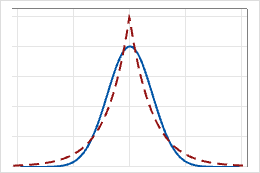# Laplace distribution

Use the Laplace distribution when the data distribution is more peaked than a normal distribution. For example, the Laplace distribution is used to model data in biology, finance, and economics applications.

The Laplace distribution is a continuous distribution that is defined by the location and scale parameters. In the following graph, the Laplace distribution is represented by the dashed line, and the normal distribution is represented by the solid line.The Laplace distribution is also known as the double exponential distribution, because it is often considered as two exponential distributions, one positive and one negative.